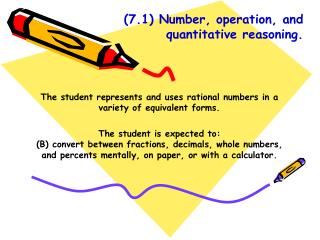# (7.1) Number, operation, and quantitative reasoning. - PowerPoint PPT PresentationDownload Presentation(7.1) Number, operation, and quantitative reasoning.

(7.1) Number, operation, and quantitative reasoning.Download Presentation## (7.1) Number, operation, and quantitative reasoning.

- - - - - - - - - - - - - - - - - - - - - - - - - - - E N D - - - - - - - - - - - - - - - - - - - - - - - - - - -
##### Presentation Transcript

1. (7.1) Number, operation, and quantitative reasoning. The student represents and uses rational numbers in a variety of equivalent forms. The student is expected to: (B) convert between fractions, decimals, whole numbers, and percents mentally, on paper, or with a calculator.

2. (7.1) Number, operation, and quantitative reasoning. The student represents and uses rational numbers in a variety of equivalent forms. The student is expected to: (C) represent squares and square roots using geometric models.

3. (7.2) Number, operation, and quantitative reasoning. The student adds, subtracts, multiplies or divides to solve problems and justify solutions. The student is expected to: (B) use addition, subtraction, multiplication, and division to solve problems involving fractions and decimals.

4. (7.3) Patterns, relationships, and algebraic thinking. The student solves problems involving proportional relationships. The student is expected to: (B) estimate and find solutions to application problems involving proportional relationships such as similarity, scaling, unit costs, and related measurement units.

5. (7.4) Patterns, relationships, and algebraic thinking. The student represents a relationship in numerical, geometric, verbal, and symbolic form. • The student is expected to: • generate formulas involving conversions, perimeter, area, circumference, volume, and scaling. • graph data to demonstrate relationships in familiar concepts such as conversion, perimeter, area, circumference, volume, and scaling.

6. (7.6) Geometry and spatial reasoning. The student compares and classifies shapes and solids using geometric vocabulary and properties. • The student is expected to: • use angle measurements to classify pairs of angles as complementary or supplementary.